Part of the foundation of mathematics, Russell's paradox (also known as Russell's antinomy), discovered by Bertrand Russell in 1901, showed that the naive set theory of Frege leads to a contradiction.

Consider the set R of all sets that do not contain themselves as members. In set-theoretic notation: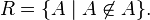$R=\{A\mid A\not\in A\}.$

Assume, as in Frege's Grundgesetze der Arithmetik, that sets can be freely defined by any condition. Then R is a well-defined set. The problem arises when it is considered whether R is an element of itself. If R is an element of R, then according to the definition, R is not an element of R; if R is not an element of R, then R has to be an element of R, again by its very definition: Hence a contradiction.

Russell's paradox was a primary motivation for the development of set theories with a more elaborate axiomatic basis than simple extensionality and unlimited set abstraction. The paradox drove Russell to develop type theory and Ernst Zermelo to develop an axiomatic set theory, which evolved into the now-canonical Zermelo–Fraenkel set theory.

### Informal presentation

An informal explanation of Russell's paradox may be given in the following way. A set can be called "normal" if it does not contain itself as a member. For example, take the set of all squares. That set is not itself a square, and therefore is not a member of the set of all squares. So it is "normal." On the other hand, if one takes the complementary set of all non-squares, that set is itself not a square and so should be one of its own members. It is "abnormal."

Now consider the set of all normal sets—give it the name R—and ask the question: Is R a "normal" set? If it is "normal," then it is a member of R, since R contains all "normal" sets. But if that is the case, then R contains itself as a member, and therefore is "abnormal." On the other hand, if R is "abnormal," then it is not a member of R, since R contains only "normal" sets. But if that is the case, then R does not contains itself as a member, and therefore is "normal." Clearly, this is a paradox: If one supposes R is "normal," one can prove it is "abnormal," and one we supposes R is "abnormal," one can prove it is "normal." Hence, R is neither "normal" nor "abnormal," which is a contradiction.

### Formal presentation

More formally, the paradox is expressed as follows. The following derivation of the paradox  reveals that the paradox requires nothing more than first-order logic with the unrestricted use of set abstraction.

Definition: The set$\{x : \Phi(x)\}\,\!$, in which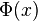$\Phi(x)\,\!$ is any predicate of first-order logic in which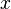$x\,\!$ is a free variable, denotes the set$A\,\!$ satisfying$\forall x\,[x \in A \leftrightarrow \Phi(x)]\,\!$.

Theorem: Defining a set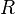$R\,\!$ by$R=\{x : x \notin x\}\,\!$ is contradictory.

Proof: Replace$\Phi(x)\,\!$ in the definition of collection with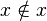$x \notin x\,\!$ and obtain for$R\,\!$ as defined: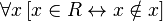$\forall x\,[x \in R \leftrightarrow x \notin x]\,\!$. Instantiating$x\,\!$ by$R\,\!$ now yields the contradiction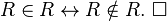$R \in R \leftrightarrow R \notin R. \ \square\,\!$

### Remark

#### Reciprocation

The force of this argument cannot be evaded by simply deeming$x \notin x\,\!$ an invalid substitution for$\Phi(x)\,\!$. In fact, there are denumerably many formulae$\Phi(x)\,\!$ giving rise to the paradox.

For example, if one takes$\Phi(x) = \neg(\exists z: x\in z\wedge z\in x)$, one gets a similar paradox; there is no set$P$ of all$x$ with this property. For convenience, refer to a set$S$ reciprocated if there is a set$T$ with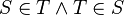$S\in T\wedge T\in S$; then$P$, the set of all non-reciprocated sets, does not exist. If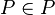$P\in P$, one would immediately have a contradiction, since$P$ is reciprocated (by itself) and so should not belong to$P$. But if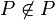$P\not\in P$, then$P$ is reciprocated by some set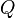$Q$, so that we have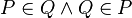$P\in Q\wedge Q\in P$, and then$Q$ is also a reciprocated set, and so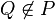$Q\not\in P$, another contradiction.

#### Independence from excluded middle

Often, as is done above, the set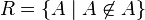$R=\{A\mid A\not\in A\}$ is shown to lead to contradiction based upon the law of excluded middle, by showing that absurdity follows from assuming$P$ true and from assuming it false. Thus, it may be tempting to think that the paradox is avoidable by avoiding the law of excluded middle, as with intuitionistic logic. However, the paradox still occurs using the law of non-contradiction:

From the definition of R, we have that RR ↔ ¬(RR). Then RR → ¬(RR) (biconditional elimination). But also RR → RR (the law of identity), so RR → (RR ∧ ¬(RR)). But, the law of non-contradiction tells us ¬(RR ∧ ¬(RR)). Therefore, by modus tollens, we conclude ¬(RR).

But since RR ↔ ¬(RR), one also has that ¬(RR) → RR, and so one also concludes RR by modus ponens. So using only intuitionistically valid methods we can still deduce both RR and its negation.

More simply, it is intuitionistically impossible for a proposition to be equivalent to its negation. Assume P ↔ ¬P. Then P → ¬P. Hence ¬P. Symmetrically, one can derive ¬¬P, using ¬P → P. So one has inferred both ¬P and its negation from our assumption, with no use of excluded middle.

## History

Exactly when Russell discovered the paradox is not known. It seems to have been May or June 1901, probably as a result of his work on Cantor's theorem that the number of entities in a certain domain is smaller than the number of subclasses of those entities. (In modern terminology, the cardinality of a set is strictly less than that of its power set.) He first mentioned the paradox in a 1901, paper in the International Monthly, entitled "Recent work in the philosophy of mathematics." He also mentioned in Cantor's proof that there is no greatest cardinal, adding that "the master" had been guilty of a subtle fallacy that he would discuss later. Russell also mentioned the paradox in his Principles of Mathematics (not to be confused with the later Principia Mathematica), calling it "The Contradiction." Again, he said that he was led to it by analyzing Cantor's "no greatest cardinal" proof.

Famously, Russell wrote to Frege about the paradox in June 1902, just as Frege was preparing the second volume of his Grundgesetze der Arithmetik. Frege hurriedly wrote an appendix admitting to the paradox, and proposed a solution that was later proved unsatisfactory. In any event, after publishing the second volume of the Grundgesetze, Frege wrote little on mathematical logic and the philosophy of mathematics.

Zermelo, while working on the axiomatic set theory he published in 1908, also noticed the paradox but thought it beneath notice, and so never published anything about it. Zermelo's system avoids the paradox thanks to replacing arbitrary set comprehension with weaker existence axioms, such as his axiom of separation (Aussonderung).

Russell and Alfred North Whitehead wrote the three volumes of Principia Mathematica (PM) hoping to succeed where Frege had failed. They sought to banish the paradoxes of naive set theory by employing a theory of types they devised for this purpose. While they succeeded in grounding arithmetic in a fashion, it is not at all evident that they did so by logic alone. In any event, Kurt Gödel in 1930-31, proved that the logic of much of PM, now known as first order logic, is complete, but that Peano arithmetic is necessarily incomplete if it is consistent. There and then, the logicist program of Frege-PM died.

## Applied versions

There are some versions of this paradox that are closer to real-life situations and may be easier to understand for non-logicians. For example, the Barber paradox supposes a barber who shaves men if and only if they do not shave themselves. When one thinks about whether the barber should shave himself or not, the paradox begins to emerge.

As another example, consider five lists of encyclopedia entries within the same encyclopedia:

 List of articles about people: Ptolemy VII of Egypt Hermann Hesse Don Nix Don Knotts Biography of Nikola Tesla Sherlock Holmes Emperor Kōnin List of articles starting with the letter L: L L!VE TV L&H ... List of articles starting with the letter K List of articles starting with the letter L List of articles starting with the letter M ... List of articles about places: Leivonmäki Katase River Enoshima List of articles about Japan: Emperor Kōnin Katase River Enoshima List of all lists that do not contain themselves: List of articles about Japan List of articles about places List of articles about people ... List of articles starting with the letter K List of articles starting with the letter M ... List of all lists that do not contain themselves?

If the "List of all lists that do not contain themselves" contains itself, then it does not belong to itself and should be removed. However, if it does not list itself, then it should be added to itself.

While appealing, these layman's versions of the paradox share a drawback: An easy refutation of the Barber paradox seems to be that such a barber does not exist. The whole point of Russell's paradox is that the answer "such a set does not exist" means the definition of the notion of set within Frege's system is unsatisfactory. This motivated the investigation of axiomatic set theory that does not suffer from the paradox of the kind.

## Set-theoretic responses

Russell, together with Alfred North Whitehead, sought to banish the paradox by developing type theory. The culmination of this research is the work, Principia Mathematica. While Principia Mathematica avoided the known paradoxes and allows the derivation of a great deal of mathematics, other challenges to predominant set theory arose.

In 1908, Ernst Zermelo proposed an axiomatization of set theory that avoided Russell's and other related paradoxes. Modifications to this axiomatic theory proposed in the 1920s, by Abraham Fraenkel, Thoralf Skolem, and by Zermelo himself resulted in the axiomatic set theory called ZFC. This theory became widely accepted once Zermelo's axiom of choice ceased to be controversial, and ZFC has remained the canonical axiomatic set theory down to the present day. ZFC does not assume that, for every property, there is a set of all things satisfying that property. Rather, it asserts that given any set X, any subset of X definable using first order logic exists. The object R discussed above cannot be constructed in this fashion, and is therefore not a ZFC set. In some extensions of ZFC, objects like R are called proper classes. ZFC is silent about types, although some contend that Zermelo's axioms tacitly presupposes a background type theory.

Through the work of Zermelo and others, especially John von Neumann, the structure of what some see as the "natural" objects described by ZFC eventually became clear; they are the elements of the von Neumann universe, V, built up from the empty set by transfinitely iterating the power set operation. It is thus now possible again to reason about sets in a non-axiomatic fashion without running afoul of Russell's paradox, namely by reasoning about the elements of V. Whether it is appropriate to think of sets in this way is a point of contention among the rival points of view on the philosophy of mathematics.

Other resolutions to Russell's paradox, more in the spirit of type theory, include the axiomatic set theories New Foundations (by Quine) and Scott-Potter set theory.